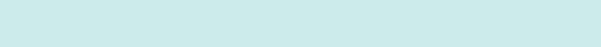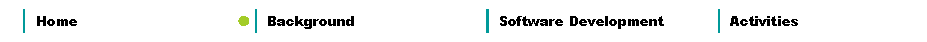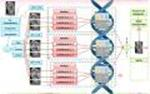IEEE Computational Intelligence SocietyNeural Network Technical Committee
 Task Force on Hybrid Intelligent Systems
 Background Soft computing can be used to build hybrid intelligent systems for achieving different goals in real-world applications. Soft Computing (SC) techniques include, at the moment, fuzzy logic, neural networks, genetic algorithms, chaos theory methods, and other similar techniques that have been proposed in recent years. Each of these techniques has advantages and disadvantages, and several real-world problems have been solved, by using one of these techniques. However, many real-world complex problems require the integration of several of these techniques to really achieve the efficiency and accuracy needed in practice. In particular, genetic algorithms can be used to optimize the topology of a fuzzy or a neural system. Also, there are neuro-fuzzy approaches or even neuro-fuzzy-genetic approaches for designing the best intelligent system for a particular application. The Task force will consider different combination of SC methodologies to design efficient hybrid intelligent systems. Neural networks are computational models with learning (or adaptive) characteristics that model the human brain. Generally speaking, biological natural neural networks consist of neurons and connections between them, and this is modeled by a graph with nodes and arcs to form the computational neural network. This graph along with a computational algorithm to specify the learning capabilities of the system is what makes the neural network a powerful methodology to simulate intelligent or expert behavior. Neural networks can be classified in supervised and unsupervised. The main difference is that in the case of the supervised neural networks the learning algorithm uses input-output training data to model the dynamic system, on the other hand, in the case of unsupervised neural networks only the input data is given. In the case of an unsupervised network, the input data is used to make representative clusters of all the data. It has been shown, that neural networks are universal approximators, in the sense that they can model any general function to a specified accuracy and for this reason neural networks have been applied to problems of system identification, control, diagnosis, time series prediction, and pattern recognition. The complexity of real-world problems has also motivated the introduction of modular and ensemble neural networks. In this case, of particular interest is the problem of response integration, which is very important because response integration is responsible for combining all the outputs of the modules. Basically, a modular or ensemble neural network uses several monolithic neural networks to solve a specific problem. The basic idea is that combining the results of several simple neural networks we can achieve a better overall result in terms of accuracy and also learning can be done faster. For example, for pattern recognition problems, which have great complexity and are defined over high dimensional spaces, modular neural networks are a great alternative for achieving the level of accuracy and efficiency needed for real-time applications. Fuzzy logic is an area of soft computing that enables a computer system to reason with uncertainty. A fuzzy inference system consists of a set of if-then rules defined over fuzzy sets. Fuzzy sets generalize the concept of a traditional set by allowing the membership degree to be any value between 0 and 1. This corresponds, in the real world, to many situations where it is difficult to decide in an unambiguous manner if something belongs or not to a specific class. Fuzzy expert systems, for example, have been applied with some success to problems of decision, control, diagnosis and classification, just because they can manage the complex expert reasoning involved in these areas of application. The main disadvantage of fuzzy systems is that they can't adapt to changing situations. For this reason, it is a good idea to combine fuzzy logic with neural networks or genetic algorithms, because either one of these last two methodologies could give adaptability to the fuzzy system. On the other hand, the knowledge that is used to build these fuzzy rules is uncertain. Such uncertainty leads to rules whose antecedents or consequents are uncertain, which translates into uncertain antecedent or consequent membership functions. Type-1 fuzzy systems, like the ones mentioned above, whose membership functions are type-1 fuzzy sets, are unable to directly handle such uncertainties. In this case, type-2 fuzzy systems, in which the antecedent or consequent membership functions are type-2 fuzzy sets are better suited for modeling these problems. Such sets are fuzzy sets whose membership grades themselves are type-1 fuzzy sets; they are very useful in circumstances where it is difficult to determine an exact membership function for a fuzzy set. Another way to handle this higher degree of uncertainty is to use intuitionistic fuzzy logic, which can also be considered as a generalization of type-1 fuzzy logic. In intuitionistic fuzzy logic the uncertainty in describing fuzzy sets is modeled by using at the same time the membership function and the non-membership function of a set (assuming that they are not complementary). Genetic algorithms and evolutionary methods are optimization methodologies based on principles of nature. Both methodologies can also be viewed as searching algorithms because they explore a space using heuristics inspired by nature. Genetic algorithms are based on the ideas of evolution and the biological process that occur at the DNA level. Basically, a genetic algorithm uses a population of individuals, which are modified by using genetic operators in such a way as to eventually obtain the fittest individual. Any optimization problem has to be represented by using chromosomes, which are a codified representation of the real values of the variables in the problem. Both, genetic algorithms and evolutionary methods can be used to optimize a general objective function. As genetic algorithms are based on the ideas of natural evolution, we can use this methodology to evolve a neural network or a fuzzy system for a particular application. The problem of finding the best architecture of a neural network is very important because there are no theoretical results on this, and in many cases we are forced to trial and error unless we use a genetic algorithm to automate this process. A similar thing occurs in finding out the optimal number of rules and membership functions of a fuzzy system for a particular application, here a genetic algorithm can also help us avoid time consuming trial and error